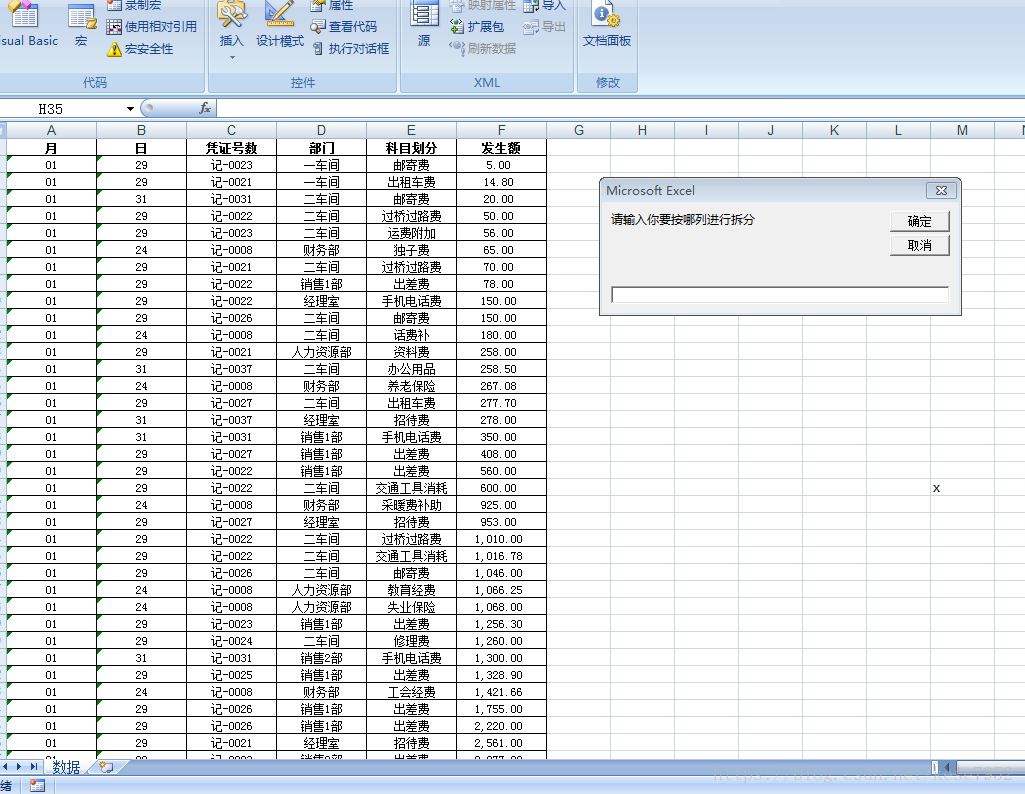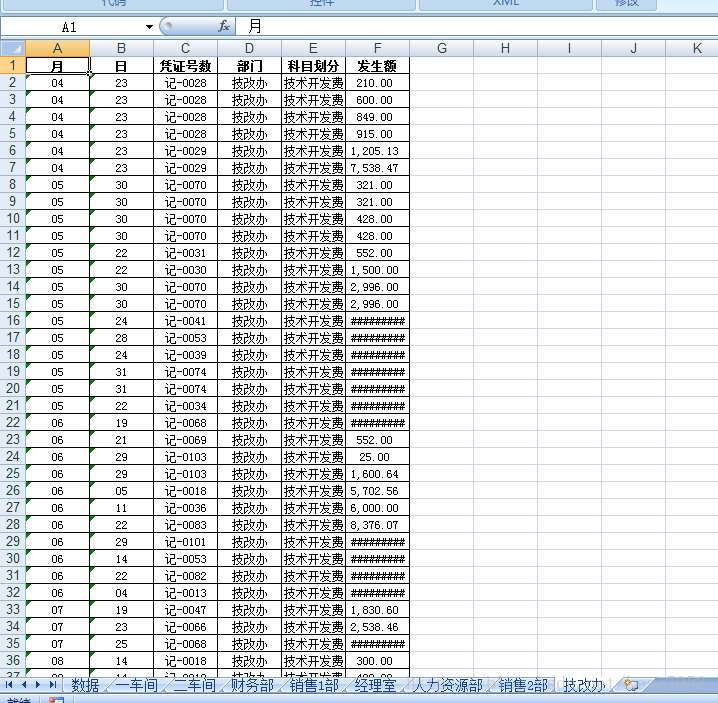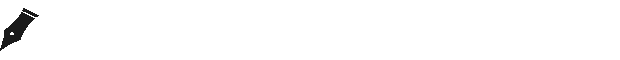0
0
01. 云栖社区>
2. 博客>
3. 正文

杨老师课堂_VBA学习教程之根据部门列创建工作表Sub 根据部门创建表并且完成数据拆分最终版()

Dim sht As Worksheet '定义变量  sht作为一个工作表对象
Dim i, k, j As Integer '定义变量 i,k,j 作为一个整数类型对象
Dim m  As Integer '定义变量 m 作为一个整数类型对象  表示 客户端输入的拆分列数
Dim irow  As Integer '定义变量 irow 作为一个整数类型对象  代表的是有效数据的最后一行

irow = Sheet1.Range("a65536").End(xlUp).Row
'删除
Application.DisplayAlerts = False '不显示删除警告框
For Each sht In Sheets          '表对象  sht 在  表集合(sheets)中进行循环

If sht.Name <> "数据" Then   '如果表的名字  不等于  数据   那么

sht.Delete              ' 表执行删除操作

End If                      '结束如果语句

Next                            ' 结束循环语句

m = InputBox("请输入你要按哪列进行拆分")  'input代表输入 ， box为盒子；  inputbox 表示：输入框  m 代表接收 客户端输入的整数类型

'根据部门建表
For i = 2 To irow   ' i 代表整数  从2到有效数据的最后进行循环
k = 0           ' k 代表一个标记   初始化时，就为0，该标记作为后面判断的条件
For Each sht In Sheets '表对象  sht 在  表集合(sheets)中进行循环

If sht.Name = Sheet1.Cells(i, m) Then '如果表的名字 等于 第一个表的单元格(行，列) 那么
k = 1                       ' 将 标记变量  k  设置为一个数字  例如：1
End If       '结束如果语句
Next      ' 结束循环语句

If k = 0 Then '如果标记的变量 k  = 0   那么  执行下列代表  但是如果不等于 则不执行
Sheets.Add after:=Sheets(Sheets.Count)  '在最后一张表后执行添加表 操作
Sheets(Sheets.Count).Name = Sheet1.Cells(i, m) ' 添加后的表的名字wie 第一个表的单元格(行，列)
End If   '结束如果语句
Next        ' 结束循环语句

'拷贝数据 ：j 代表的是表的序号
For j = 2 To Sheets.Count
' 第一个表的单元格区域为 a1 到 f 有效数据最后一行  执行筛选   筛选列为 输入的列m  条件是 表的名字(指定列名相同)
Sheet1.Range("a1：f" & irow).AutoFilter Field:=m, Criteria1:=Sheets(j).Name
' 第一个表的单元格区域为 a1 到 f 有效数据最后一行执行拷贝  到  循环到的某张表的a1单元格
Sheet1.Range("a1：f" & irow).Copy Sheets(j).Range("a1")
' 第一个表的单元格区域为 a1 到 f 有效数据最后一行 执行筛选(取消筛选操作)
Sheet1.Range("a1：f" & irow).AutoFilter
Next ' 结束循环语句
End Sub+ 关注

corcosa 13924人浏览